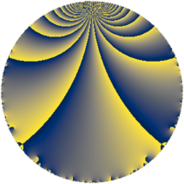# Properties

 Label 70.2.cLevel $70$ Weight $2$ Character orbit 70.c Rep. character $\chi_{70}(29,\cdot)$ Character field $\Q$ Dimension $4$ Newform subspaces $1$ Sturm bound $24$ Trace bound $0$

# Related objects

## Defining parameters

 Level: $$N$$ $$=$$ $$70 = 2 \cdot 5 \cdot 7$$ Weight: $$k$$ $$=$$ $$2$$ Character orbit: $$[\chi]$$ $$=$$ 70.c (of order $$2$$ and degree $$1$$) Character conductor: $$\operatorname{cond}(\chi)$$ $$=$$ $$5$$ Character field: $$\Q$$ Newform subspaces: $$1$$ Sturm bound: $$24$$ Trace bound: $$0$$

## Dimensions

The following table gives the dimensions of various subspaces of $$M_{2}(70, [\chi])$$.

Total New Old
Modular forms 16 4 12
Cusp forms 8 4 4
Eisenstein series 8 0 8

## Trace form

 $$4 q - 4 q^{4} + 4 q^{5} - 12 q^{9} + O(q^{10})$$ $$4 q - 4 q^{4} + 4 q^{5} - 12 q^{9} + 4 q^{10} - 4 q^{14} + 12 q^{15} + 4 q^{16} - 16 q^{19} - 4 q^{20} - 8 q^{26} - 8 q^{29} + 12 q^{30} + 16 q^{31} + 8 q^{34} + 4 q^{35} + 12 q^{36} + 24 q^{39} - 4 q^{40} - 24 q^{41} - 12 q^{45} + 8 q^{46} - 4 q^{49} - 4 q^{50} - 24 q^{55} + 4 q^{56} + 16 q^{59} - 12 q^{60} + 24 q^{61} - 4 q^{64} - 4 q^{65} - 48 q^{66} + 48 q^{69} - 4 q^{70} - 24 q^{71} + 8 q^{74} + 16 q^{76} - 8 q^{79} + 4 q^{80} - 36 q^{81} - 8 q^{85} - 16 q^{86} + 40 q^{89} - 12 q^{90} - 8 q^{91} + 16 q^{94} - 4 q^{95} + O(q^{100})$$

## Decomposition of $$S_{2}^{\mathrm{new}}(70, [\chi])$$ into newform subspaces

Label Dim $A$ Field CM Traces $q$-expansion
$a_{2}$ $a_{3}$ $a_{5}$ $a_{7}$
70.2.c.a $4$ $0.559$ $$\Q(i, \sqrt{6})$$ None $$0$$ $$0$$ $$4$$ $$0$$ $$q+\beta _{2}q^{2}+(\beta _{1}+\beta _{3})q^{3}-q^{4}+(1-\beta _{1}+\cdots)q^{5}+\cdots$$

## Decomposition of $$S_{2}^{\mathrm{old}}(70, [\chi])$$ into lower level spaces

$$S_{2}^{\mathrm{old}}(70, [\chi]) \cong$$ $$S_{2}^{\mathrm{new}}(35, [\chi])$$$$^{\oplus 2}$$